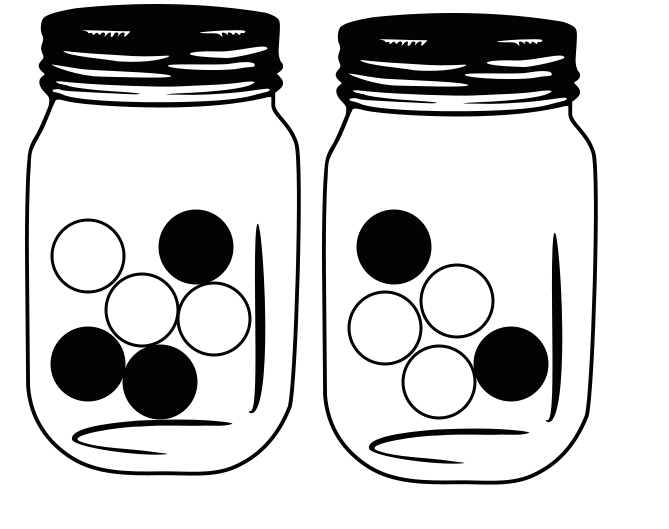# Pick White Marble

Probability Level 5You have 50 white marbles and 50 black marbles, and you are allowed to distribute them as you please in two jars. Then you are blindfolded, pick a jar at random, and a marble from that jar.

Over all possible distributions of the marbles in the two jars, suppose that the maximum value of the probability that the marble picked is white is $\dfrac{m}{n}$, where $m,n$ are coprime positive integers. Find $m+n$.

×